# ISEE Lower Level Quantitative : How to find the area of a square

## Example Questions

### Example Question #1 : How To Find The Area Of A Square

What is the area of a square if one side of the square is 6?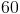Explanation:

If one side of a square is 6, then each of the four sides of the square are equal to 6. To find the area of a square, we multiply the length and the height together. The length is 6, and the height is 6, thus the equation we use is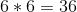.

Remember the formula for the area of a quadrilateral is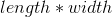. For a square, one side is equal to both the length and the width.

### Example Question #396 : Quadrilaterals

One side of a square iscentimeters long. What is the area of the square?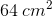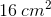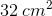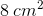Explanation:

The formula for finding the area of a square is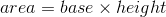, or, because this is a square,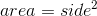.

area =centimeters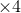centimeters, or### Example Question #397 : Quadrilaterals

A right triangle has a base ofand a height of.

What is the area of the rectangle made by 2 of these triangles aligned along the hypotenuse?Explanation:

If one combines the 2 identical triangles, their base and height become the length and width of the rectangle.

Area of a rectangle is: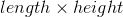In this case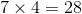### Example Question #398 : Quadrilaterals

A square has an area of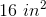.  What is the length of one side?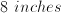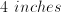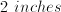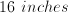Explanation:

You can find the area of a square by multiplying two sides together.  All of the sides of a square are equal.  In this case,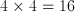, so the length of all of the sides of the square is 4 inches.

### Example Question #399 : Quadrilaterals

Michaela drew th square below.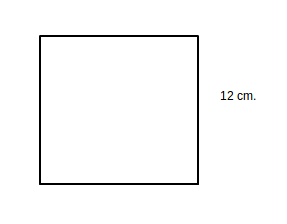What is the area of the square?

48 square centimeters

12 square centimeters

100 square centimeters

112 square centimeters

144 square centimeters

144 square centimeters

Explanation:

The area of a square can be found by multiplying the length of a side times itself.  The side length of the above square is 12 cm. By finding 12 x 12, we find that the area of the square is 144 cm. squared.

### Example Question #2 : How To Find The Area Of A Square

James found the area of the square below to be 36 centimeters squared.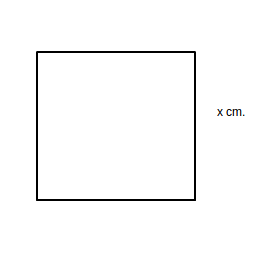What is the length of one side of the square?

6 centimeters

36 centimeters

8 centimeters

18 centimeters

9 centimeters

6 centimeters

Explanation:

The area of a square can be found by multiplying the length of a side by itself.  36 is equal to 6 times 6, therefore the length of one side is 6 centimeters.

### Example Question #3 : How To Find The Area Of A Square

Daphne found the area of the square below to be 81 centimeters squared.What is the length of one side of the square?

8 centimeters

7 centimeters

81 centimeters

20 centimeters

9 centimeters

9 centimeters

Explanation:

The area of a square can be found by multiplying the length of a side by itself.  81 is equal to 9 times 9, therefore the length of one side is 9 centimeters.

### Example Question #4 : How To Find The Area Of A Square

If the area of a square is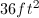, what is the length of each side?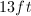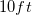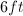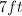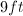To find the area of a square, the length must be multiplied by the height.  Since a square has four equal sides, the length and width will have the same measurement. We must, therefore, find the square root of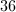.  Since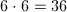,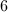is the square root of, which makes it the measurement for both the length and the width of the square.